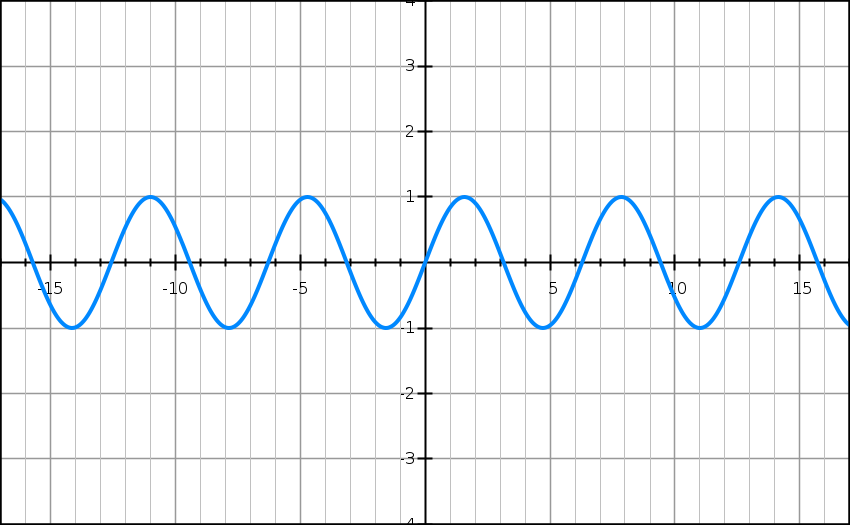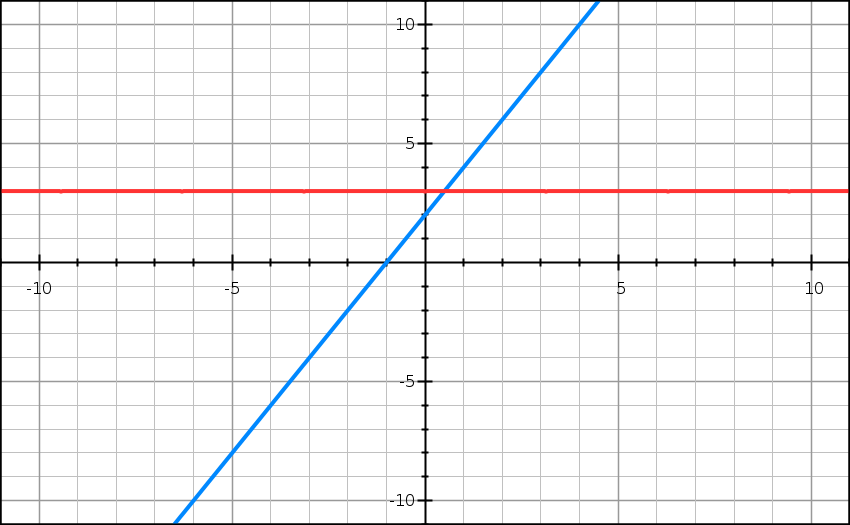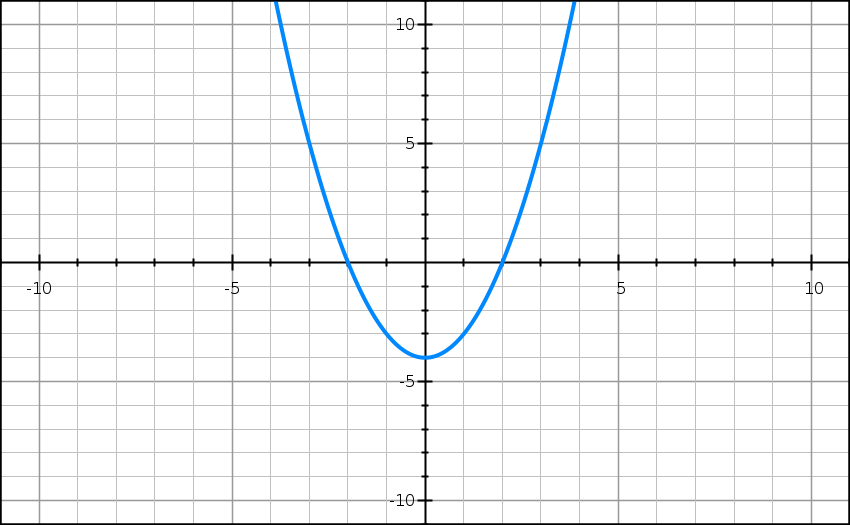# Graph Simple Equations

### GraphSketch

The old equation grapher we provided used Flash, which is grossly out-of-date and not widely supported any longer. We're hoping to bring back a new tool to graph equations, but for the moment, we'd recommend GraphSketch, which is a free tool to produce nice multi-function graphs. You can also save the images for use elsewhere. Here are some example graphs to show what you can do:

$$f(x)=sin(x)$$$$f(x)=2x+2$$ and $$g(x)=3$$$$f(x)=x^2-4$$Our old tool is down, but try Graph Sketch instead!